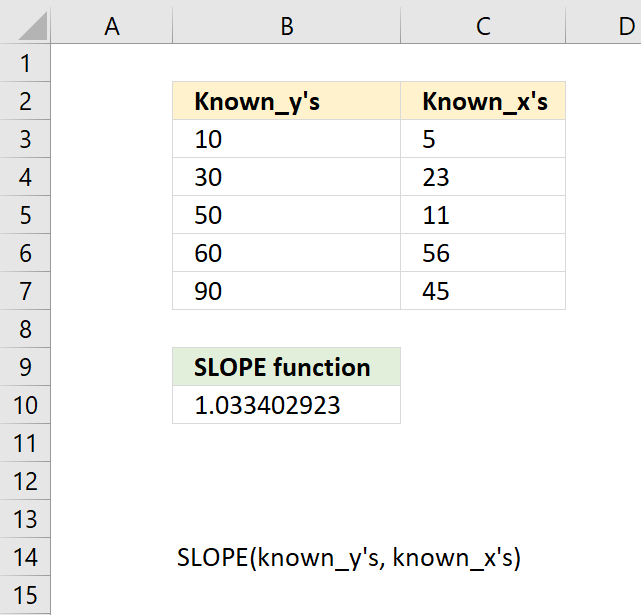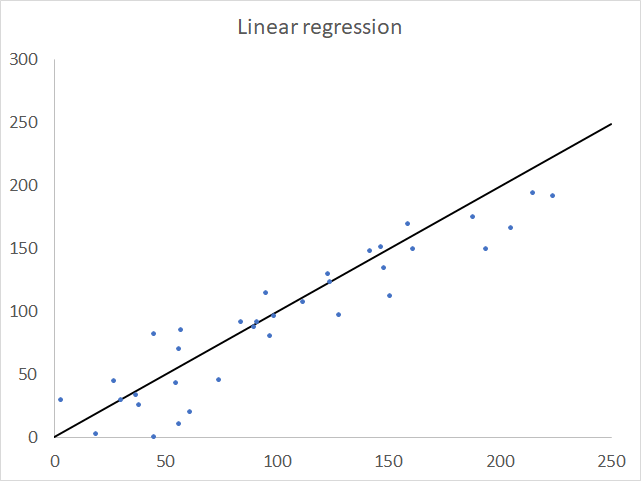Author: Oscar Cronquist Article last updated on August 28, 2018The SLOPE function calculates the slope of the linear regression line through coordinates.

Formula in cell B10:

=SLOPE(B3:B7,C3:C7)

### Excel Function Syntax

SLOPE(known_y's, known_x's)

### Arguments

 known_y's Required. An array or cell reference to dependent data points. known_x's Required. An array or cell reference to independent data points.

Zero values are included but text, logical values and empty cells are ignored.

The SLOPE function returns

• #N/A! error if there are a different number of values in known_y's and known_x's.A linear regression line is an average of a given set of coordinates and assumes there is a relationship between them.

### Formula equation